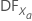Methods and formulas for stepwise in Analyze Factorial Design

Select the method or formula of your choice.

Forward information criteria procedure

A method for determining which variables to retain in a model. The forward information criteria procedure adds the term with the lowest p-value to the model at each step. Additional terms can enter the model in 1 step if the settings for the analysis allow consideration of non-hierarchical terms but require each model to be hierarchical. Minitab calculates the information criteria for each step. Minitab displays the results of the analysis for the model with the minimum value of the selected information criterion, either AICc or BIC. In most cases, the procedure continues until one of the following conditions occurs:
• The procedure does not find a new minimum of the criterion for 8 consecutive steps.
• The procedure fits the full model.
• The procedure fits a model that leaves 1 degree of freedom for error.
If you specify settings for the procedure that require a hierarchical model at each step and allow only one term to enter at a time, then the procedure continues until it either fits the full model or fits a model that leaves 1 degree of freedom for error. Minitab displays the results of the analysis for the model with the minimum value of the selected information criterion, either AICc or BIC.

Forward selection procedure

A method for determining which terms to retain in a model. Forward selection adds variables to the model using the same method as the stepwise procedure. Once added, a variable is never removed. The default forward selection procedure ends when none of the candidate variables have a p-value smaller than the value specified in Alpha to enter.

Stepwise method

Performs variable selection by adding or deleting predictors from the existing model based on the F-test. Stepwise is a combination of forward selection and backward elimination procedures.

If the initial model uses all of the degrees of freedom, the analysis for factorial designs does not stop as other analyses in Minitab do. Instead, with Analyze Factorial Design and Analyze Variability, Minitab removes ¼ of the terms to obtain sufficient degrees of freedom to begin. The number of terms to remove is rounded to the nearest integer and has a maximum of 9. From the saturated model, Minitab removes the terms with the smallest adjusted sums of squares while maintaining model hierarchy. Minitab does not reconsider these terms at later steps. The table of model selection details lists the terms that Minitab removed.

Variables to remove

Minitab calculates an F-statistic and p-value for each variable in the model. If the model contains j variables, then F for any variable, xr , is this formula:

Notation

TermDescription
SSE(jXr ) SS Error for the model that does not contain xr
SSE j SS Error for the model that contains xr
MSE j MS Error for the model that contains xr

If the p-value for any variable is greater than the value specified in Alpha to remove, then Minitab removes the variable with the largest p-value from the model, calculates the regression equation, displays the results, and initiates the next step.

If Minitab cannot remove a variable, the procedure attempts to add a variable. Minitab calculates an F-statistic and p-value for each variable that is not in the model. If the model contains j variables, then F for any variable, xa, is this formula:

Notation

TermDescription
SSE j SS Error before xa is added to the model
SSE(j + Xa ) SS Error after xa is added to the modelDegrees of freedom for variable Xa
MSE(j + Xa ) MS Error after xa is added to the model

If the p-value corresponding to the F-statistic for any variable is smaller than the value specified in Alpha to enter, Minitab adds the variable with the smallest p-value to the model, calculates the regression equation, displays the results, then goes to a new step. When no more variables can be entered into or removed from the model, the stepwise procedure ends.

Backward elimination procedure

A method for determining which variables to retain in a model. Backward elimination starts with the model that contains all the terms and then removes terms, one at a time, using the same method as the stepwise procedure. No variable can re-enter the model. The default backward elimination procedure ends when none of the variables included in the model have a p-value greater than the value specified in Alpha to remove.

If the initial model uses all of the degrees of freedom, the analysis for factorial designs does not stop as other analyses in Minitab do. Instead, with Analyze Factorial Design and Analyze Variability, Minitab removes ¼ of the terms to obtain sufficient degrees of freedom to begin. The number of terms to remove is rounded to the nearest integer and has a maximum of 9. From the saturated model, Minitab removes the terms with the smallest adjusted sums of squares while maintaining model hierarchy. Minitab does not reconsider these terms at later steps. The table of model selection details lists the terms that Minitab removed.

By using this site you agree to the use of cookies for analytics and personalized content.  Read our policy